Next: Magnetohydrostatic Configuration Up: Hydrostatic Equilibrium Previous: Hydrostatic Equilibrium   Contents

# Polytrope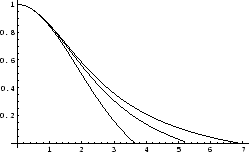If we choose the polytropic equation of stateC.1,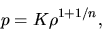(C.1)

the hydrostatic balance is expressed by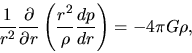(C.2)

where we used equations (4.1) and (4.2). A hydrostatic gaeous star composed with a polytropic gas is called polytrope. Normalizing the density, pressure and radius as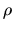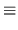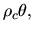(C.3)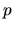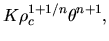(C.4)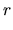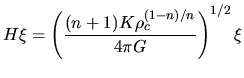(C.5)

we obtain a normalized equation as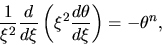(C.6)

which is called Lane-Emden equation of index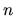. The boundary condition at the center of polytrope should be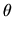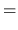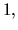(C.7)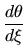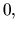(C.8)

at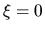. Solution of this equation is plotted for severalin Figure C.1.

Mass of the polytrope is written down as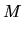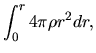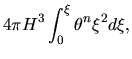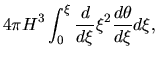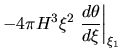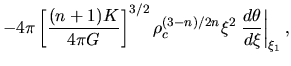(C.9)

where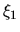represents the zero point of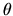or the surface radius normalized by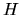. For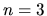or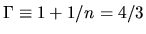, equation (C.9) reduces to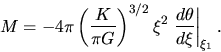(C.10)

Thus, the mass does not depend on the central density for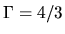polytrope. For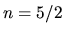or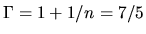,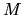is written down as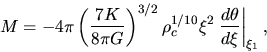(C.11)

where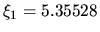and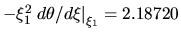. Polytrope with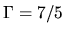gas, the mass-density relation becomes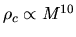. While, for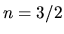of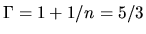,is written down as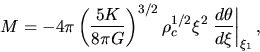(C.12)

where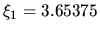and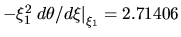.Next: Magnetohydrostatic Configuration Up: Hydrostatic Equilibrium Previous: Hydrostatic Equilibrium   Contents
Kohji Tomisaka 2007-07-08